Physics
Fox

### Summary

• An object's net force is the force acting on it in one direction, minus the force acting on it in the opposite direction.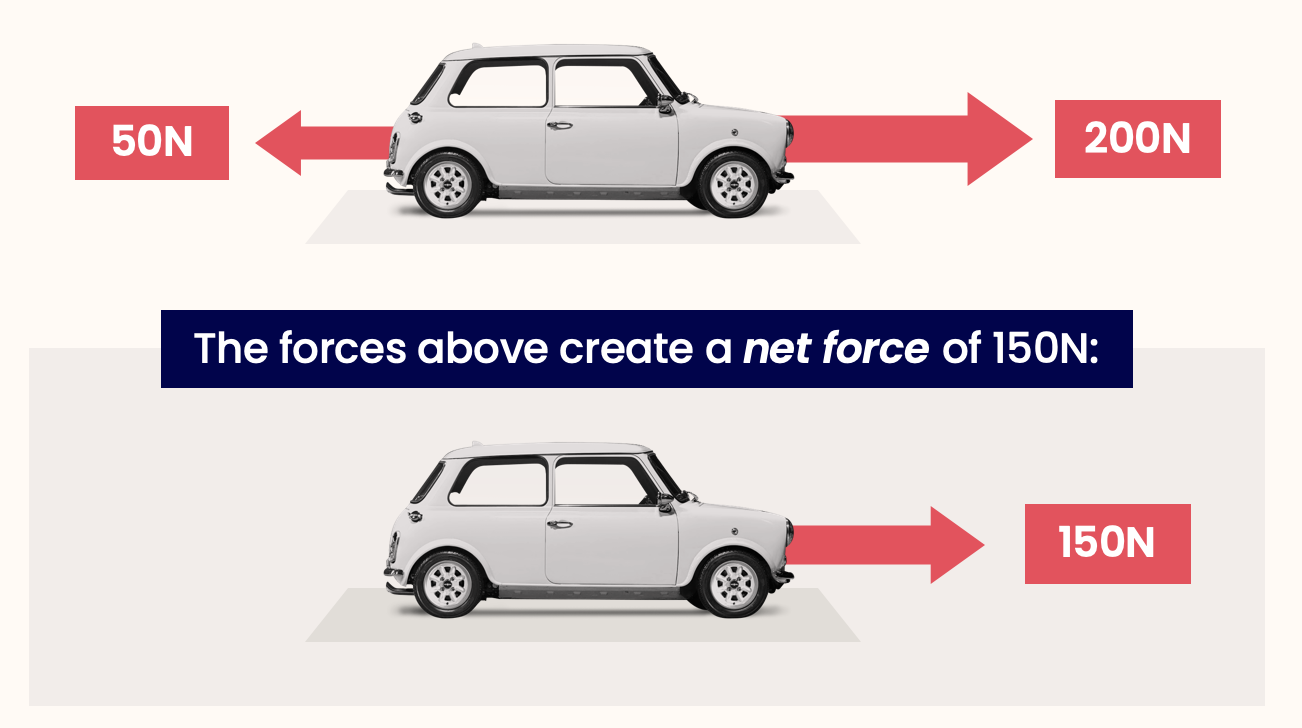Net force on this car
= 200N - 50N
= 150N

To understand the laws from the first page of this chapter, we need to know about speed, force and net force. We now know about speed and force, so all that's left to understand is net force. Let's do that now...

You might have heard of the phrase ‘net worth’: a person's net worth is how much money they own, minus how much money they owe other people.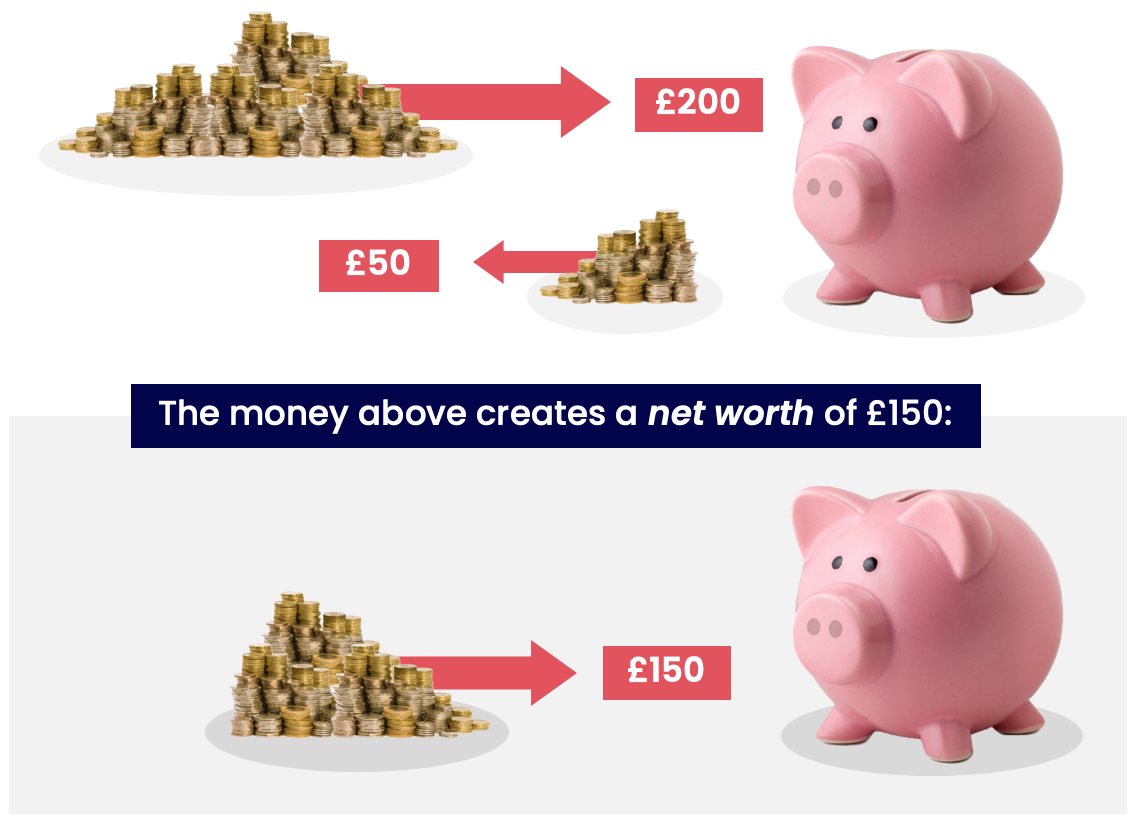For example, let's say you own £200, but you owe your friend £50. Your net worth = money owned - money owed = £200 - £50 = £150.

Net worth is important because it's the amount of money that you actually have. It doesn't matter if you own £1 million if you also owe someone else £1 million! Your net worth = £0, so it's as if you have no money at all.

Net force is a similar idea to net worth: an object's net force is the force acting on it in one direction, minus the force acting on it in the opposite direction.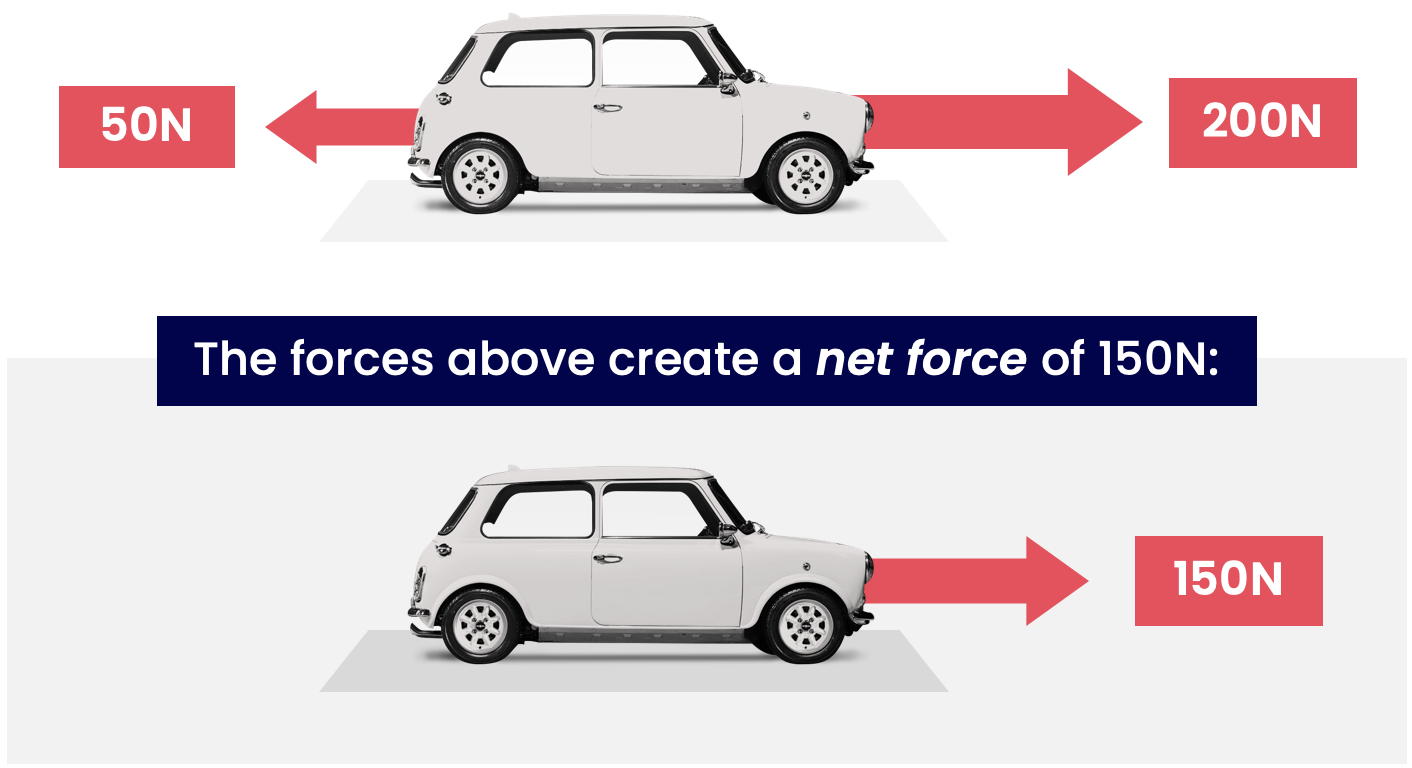For example, let's imagine the forces on a car. Let's say there's a 200N force forwards, and a 50N force backwards. The car's net force = force forwards - force backwards = 200N - 50N = 150N.

Net force is important because it's what actually makes things move. It doesn't matter if you have 1,000,000N one way if there's also 1,000,000N the other way! The net force = 0, so it's as if there's no force acting on it at all. You might see this in a tug-of-war; the rope won't move if there's an equal pull on either side — even if both pulls are very strong!

Note that force might be going upwards (e.g. for a balloon).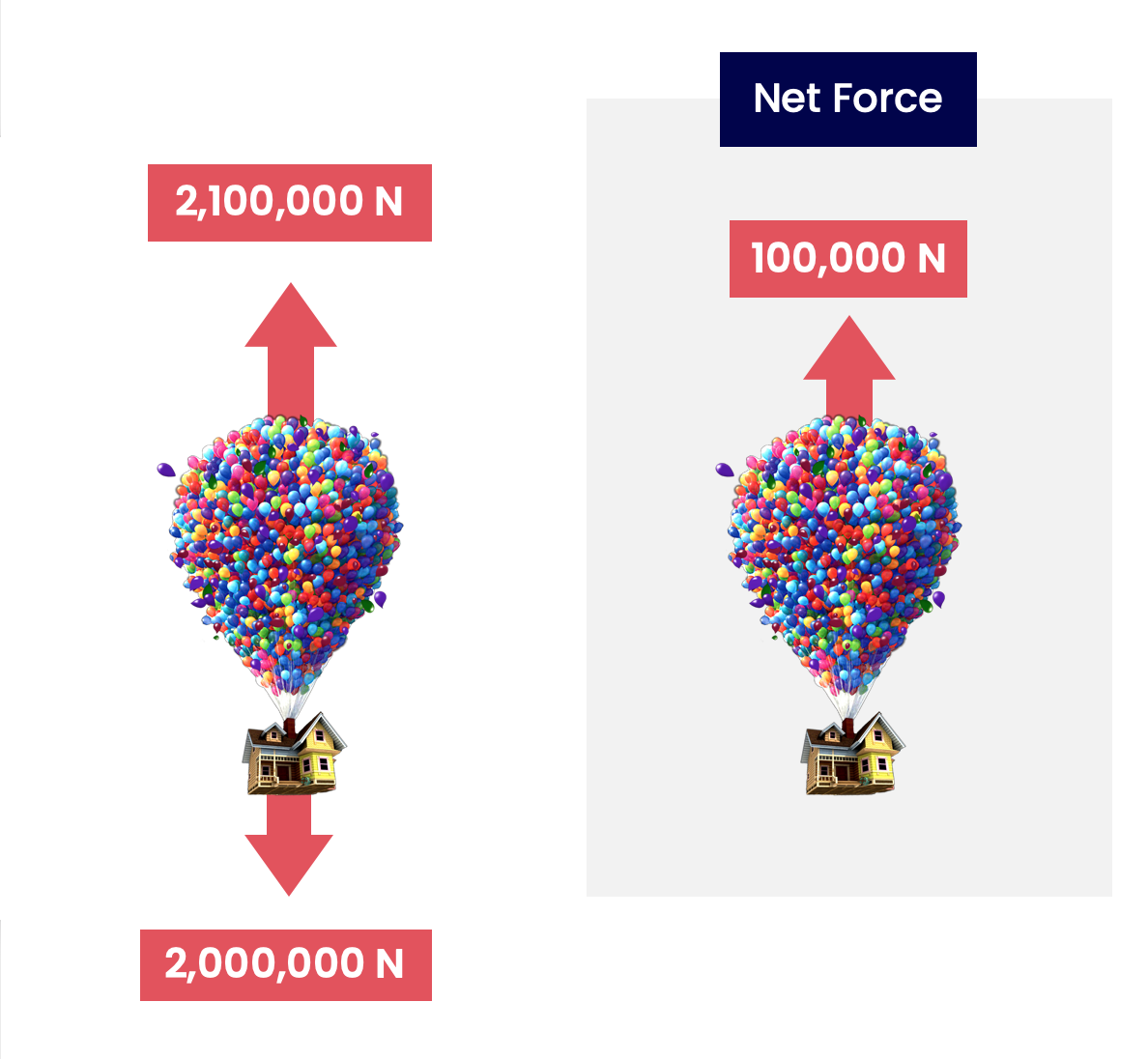The process here is the same as above; to find the net force up, we find the force up — force down.

So the net force (upwards) = 2,100,00N - 2,000,000N = 100,000N.

Or consider a falling football; to find the net force downwards, we find the force down — force up.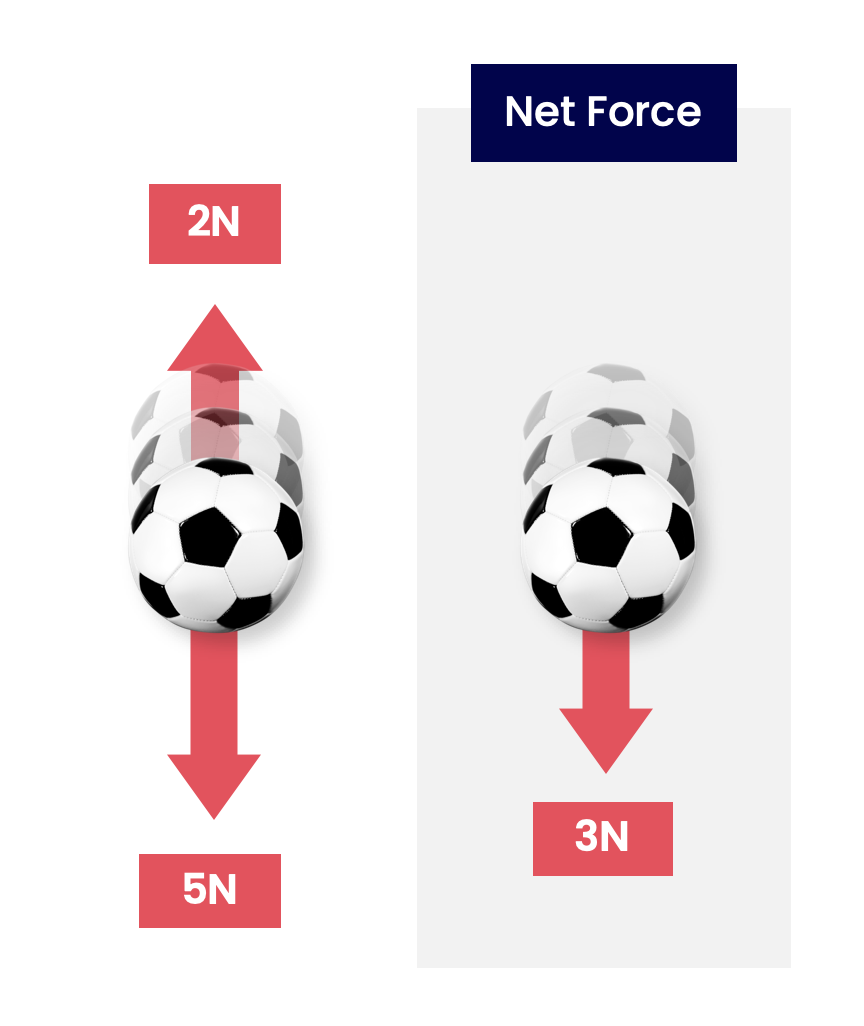So the net force (downwards) = 5N - 2N = 3N.

But what if there are forces both left-and-right, and up-and-down? We saw an example of this with a car on the previous page: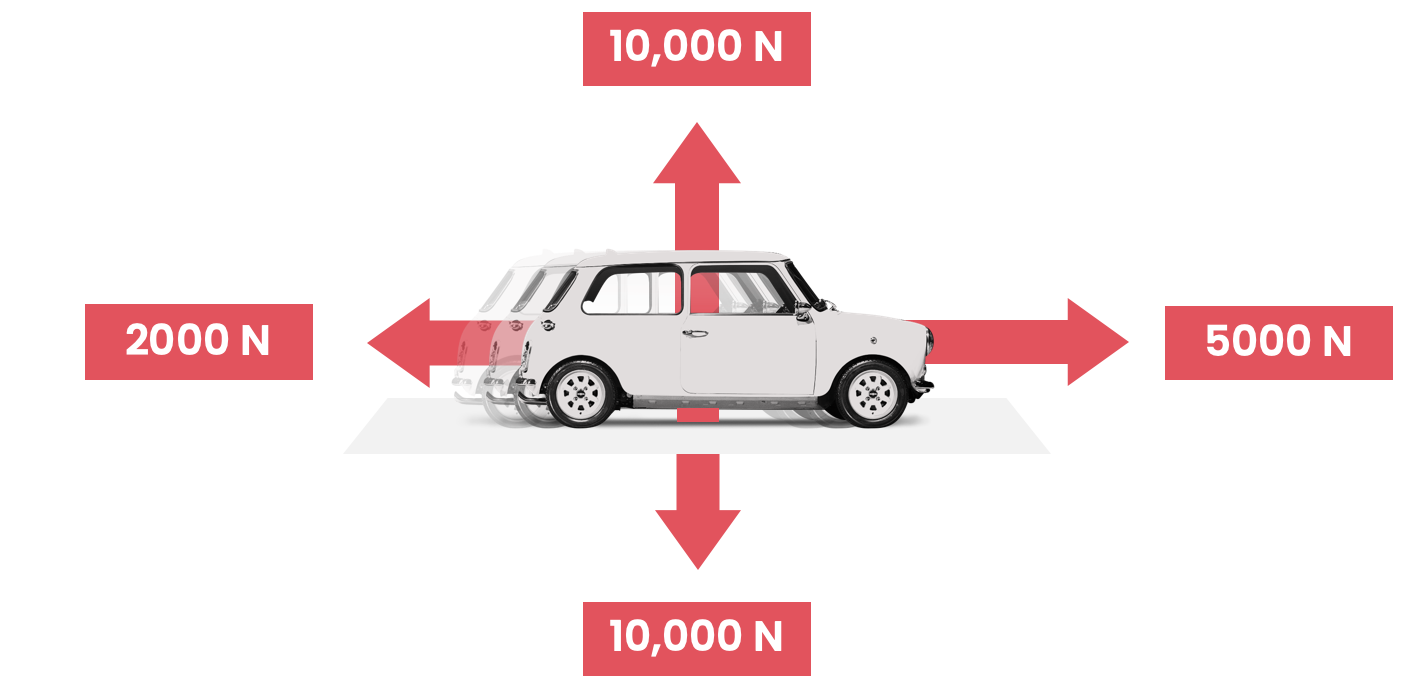We have to separately find the net force in each direction. For example, the net force right = 5000N - 2000N = 3000N. The net force upwards = 10,000N - 10,000N = 0N.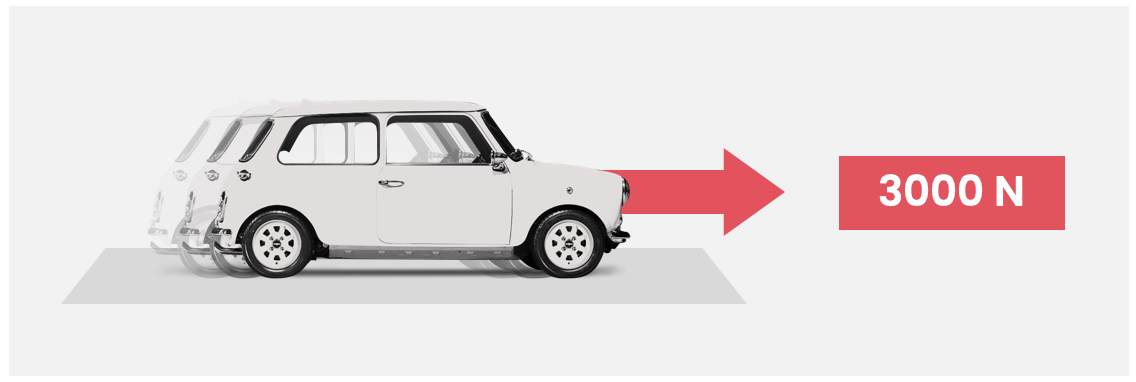So all 4 forces are equivalent to just 1 force to the right.

Quiz

### Congratulations!

6 of 6 questions completed

+ ⭐️ collected.

• You can change your display name at any time.
• at least 1 letter
• at least 1 number
• at least 8 characters